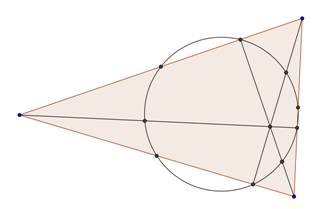The Nine Point circle or Orthiccircle, which can be constructed for any triangle, is a circle which passes through nine special points of a triangle:

• The midpoints of each side of the triangle
• The foot of each altitude
• The midpoint of the line segment from each vertex of the triangle to the orthocentre of the triangle